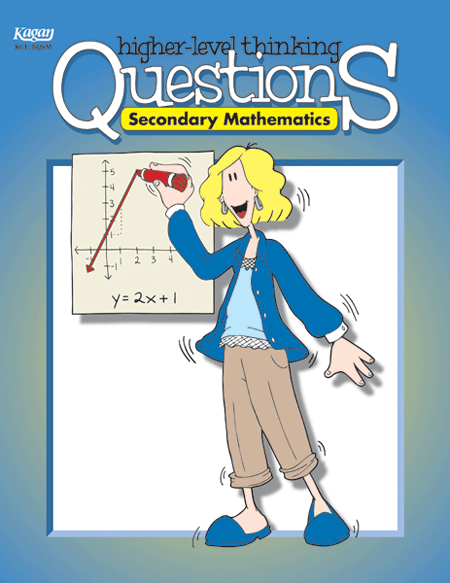## Secondary Mathematics

### Higher-Level Thinking Questions

• Hundreds of ready-to-use thinking questions on 16 Secondary Mathematics concepts, including problem solving, decimal operations, fraction concepts, Algebra, Geometry and more.
• Questions are crafted to generate critical and creative thinking in Secondary Mathematics.
• Includes reproducible prompts for journal writing and activities for student-generated questions.
• Questions are provided in convenient reproducible question card format, perfect for engaging cooperative questioning and analytical thinking.
 BQSM • \$19The mathematics standards call for moving beyond memorization, rote learning, and application of predetermined procedures. The standards call on teachers to work toward a deeper conceptual understanding and to foster mathematical reasoning. How do we foster such a deep understanding of mathematics concepts? With deep-thinking math questions, of course. You'll find questions for sixteen mathematics topics to promote mathematical thinking and interaction in your class. Question topics: Problem Solving; Number Patterns and Relationships; Whole Number and Decimal Place Value; Decimal Operations; Fraction Concepts; Fraction Addition and Subtraction; Fraction Multiplication and Division; Percents; Relating Fractions, Decimals, and Percents; Ratios, Rates, and Proportions; Statistics, Data Analysis, and Graphing; Algebra: Integers and Integer Operations; Algebra: Solving Equations and Inequalities; Geometry Concepts; Geometry and Measurement; Probability.

Sample Page
Click on the image to view and/or print it full size.

Back